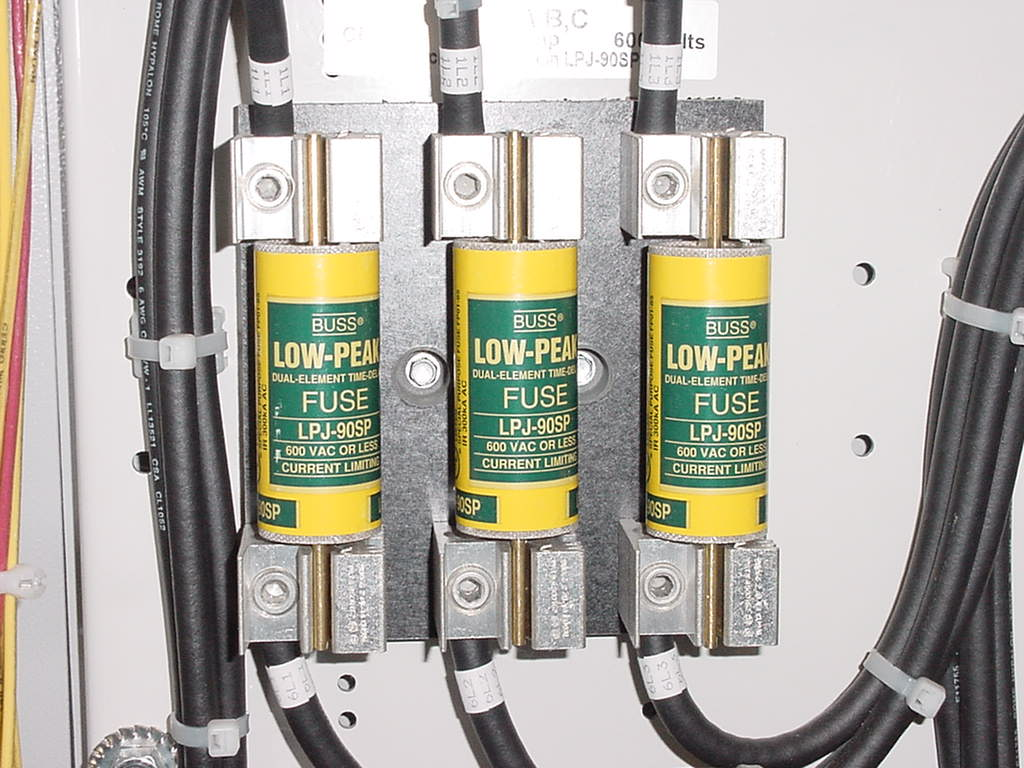Arc Flash & Electrical Power Training | Brainfiller

Arc Flash & Electrical Power Training by Jim Phillips | 800.874.8883 | +1 480.275.7451

Current Status
Not Enrolled
Price
199.00
Get Started

# Short Circuit AnalysisNational Electrical Code Section 110.9 Interrupting Ratings states: Equipment intended to interrupt current at fault levels shall have an interrupting rating at nominal circuit voltage at least equal to the current that is available at the line terminals of the equipment.

Equipment intended to interrupt current at other than fault levels shall have an interrupting rating at nominal circuit voltage at least equal to the current that must be interrupted.

How would one know whether this requirement has been met? By conducing a short circuit study to predict the available fault current at each piece of equipment.  This course takes you through the process of performing a short circuit study including the necessary data, study process and detailed short circuit calculations using Jim Phillips’ calculations worksheets which are part of this online course.

• SHORT CIRCUIT ANALYSIS – INTRODUCTION
Short Circuit Study Requirements, NEC® 110.9 and 110.10, Interrupting and Withstand Ratings, Data Requirements, Available Utility Short Circuit Current, Conductor Impedance, Source Impedance, X/R Ratio, Per Phase Calculations, Thevenin Equivalent, Impedance
• CONDUCTOR IMPEDANCE AND SHORT CIRCUIT CALCULATIONS
Determining the Source Impedance, Calculating the Conductor Impedance, Conductor Impedance Tables, Conductor Calculation Worksheets.  In Class Problems – Short Circuit Calculations with Conductor Impedance
• TRANSFORMER IMPEDANCE AND SHORT CIRCUIT CALCULATIONS
Transformer Testing and Percent Impedance, X/R Ratio,  Using Percent Impedance for Short Circuit Calculations,  Determining the Source Impedance in Percent, Infinite Bus Calculations, Transformer Calculation Worksheets.  In Class Problems – Short Circuit Calculations with Transformer Impedance
• MOTOR CONTRIBUTION
Theory of Motor Short Circuit Contribution, Sub-Transient Reactance, Xd”, Effect of Motor Contribution on Short Circuit Current, Multipliers for Motor Contribution.  In Class Problems – Consideration of Motor Contribution
• DEVICE INTERRUPTING RATINGS
Circuit Breaker and Fuse Interrupting Ratings, UL and ANSI Testing Methods, Symmetrical and Asymmetrical Short Circuit Current, Effect of X/R Ratio on Interrupting Ratings, Multiplying Factors when the X/R and Asymmetry are Too Large
• SERIES RATINGS
Development of Series Ratings, Proper Application of Series Ratings, Dynamic Impedance, Fully Rated vs. Series Rated, Current Limitation, Let Thru Current, U.L. Tests
• CASE PROBLEM
Short Circuit Study of Small Industrial System.  Calculations Include Source, Conductor and Transformer Impedance, Motor Contribution and Protective Device Adequacy Evaluation